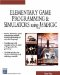# Atan

This command calculates the arctangent. Here is its syntax:

` x = acos(y) `

### Parameters

 x The angle in radians that corresponds to a tangent value of y

### Notes

The arctangent can be useful when you perform calculations that involve geometry. For example, the tangent of 45 degrees (pi/2 radians) is 1. The arctangent of 1 is then pi/2 (3.1416/2).

### Sample Code

The code below sends the angle of roll about the y-axis to the screen; this is controlled with the left and right arrow keys. The program first finds the angle, then it finds the tangent of the angle, and then it uses the atan function to find the angle again based on the tangent.

` oworld = New World(); ocamera = New Camera(oworld); sphere = oworld.CreateSphere(50,50,50,25,25); ocamera.MoveBack(1200); sphere.Walk(); //Loop While(TRUE)  {   z = sphere.getyangle();   y = tan(z);   x = atan(y);   SetText("yangle " + x );   } `Elementary Game Programming & Simulations Using Jamagic (Charles River Media Game Development)
ISBN: 1584502614
EAN: 2147483647
Year: 2002
Pages: 105
Authors: Sergio Perez